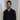# JavaScript : Value vs Reference

September 11, 2017JavaScript is a language full of surprises. One such surprise is understanding how some data types are copied by value or reference.

This can be a source of subtle bugs & confusion. So let’s try to break this down into very simple terms for those just starting out.

### Primitives

Definition

Primitives such as `Boolean`, `String`, `Number`, `null`, `undefined` are copied by value.

``````let x = 'abc';
let y = x;

console.log(x); //prints 'abc'
console.log(y); //prints 'abc'``````

In the example above, both `x` & `y` contain the value `abc` but they have separate copies of it.

### Non-primitives

Data types such as `Array`, `Object`, `Function` are copied by reference.

``````let x = ['a', 'b', 'c'];
console.log(x); //prints ['a', 'b', 'c']``````

When a variable is assigned a non-primitive value, it’s given a reference to that object’s location in memory. In this example above, the variable `x` doesn’t actually contain the value `['a', 'b', 'c']`, instead it points to a location in memory where that value is stored.

``let y = x;``

When a reference type value is copied to another variable, like `y` in the example above, the object is copied by reference instead of value. In simple terms, `x` & `y` don’t have their own copy of the value. They point to the same location in memory.

``````x.push('d');
console.log(x); //prints ['a', 'b', 'c', 'd'];
console.log(y); //prints ['a', 'b', 'c', 'd'];``````

When a new item is pushed to `x`, the array in memory is modified, and as a result the variable `y` also reflects that change.### Equality

``````const a = { year : 2017 };
const b = { year : 2017 };
const c = a;

a === b; //false
a === c; //true``````

You might expect `a===b` to resolve to true but that isn’t the case. The reason behind that is that although `a` & `b` contain identical objects, they still point to two distinct objects stored in different locations in memory.

On the contrary, `a` & `c` hold reference to the same location in memory & are therefore considered to be equal.

And there you have it. An extremely simplified & short view at how values are stored via reference vs value. If you’re a new dev & found this helpful, please drop a comment or get in touch via twitterWritten by Watandeep Sekhon.